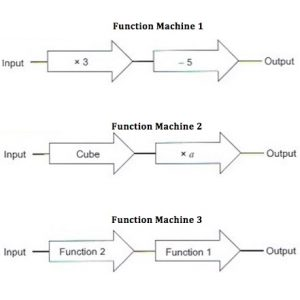The input to Function Machine 1 is x. Write down an expression for the output.

If the output is 7 what was the input?

In Function 2, a is constant. If the input is 4 what is the output?

The functions from Function Machines 1 & 2 are combined to give Function Machine 3 where the output of Function Machine 2 is used as the input for Function Machine 1.

The number 2 is put into Function Machine 3 and the output is 3. Find a.

Make up some questions of your own using these function machines.